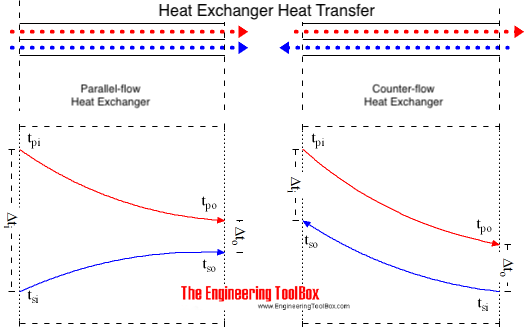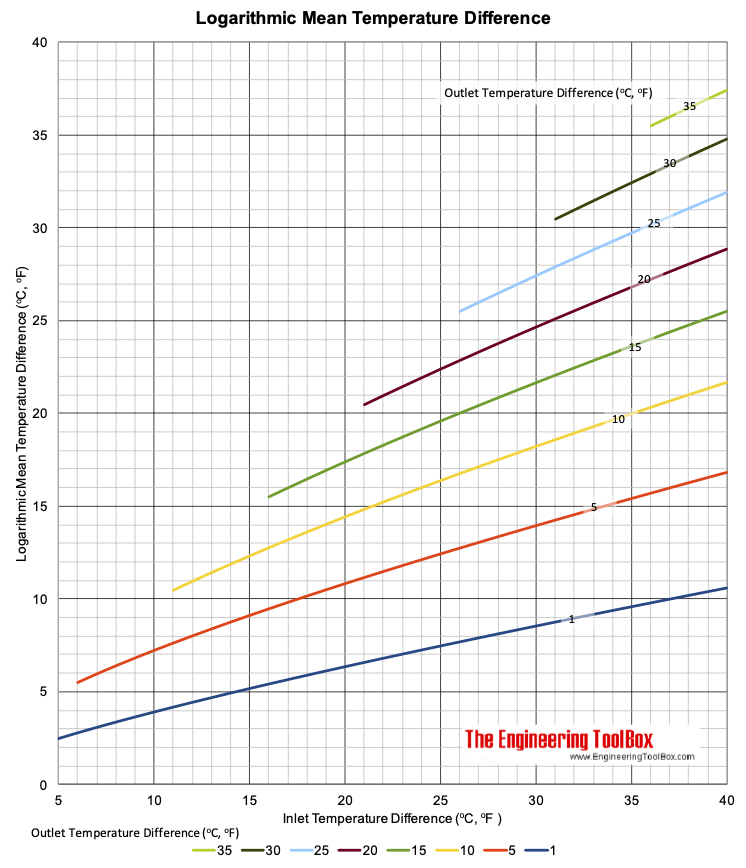Engineering ToolBox - Resources, Tools and Basic Information for Engineering and Design of Technical Applications!

# Arithmetic and Logarithmic Mean Temperature Difference

## Arithmetic Mean Temperature Difference in Heat Exchangers - AMTD - and Logarithmic Mean Temperature Difference - LMTD - formulas with examples - Online Mean Temperature Calculator.

According to Newton's Law of Cooling heat transfer rate is related to the instantaneous temperature difference between hot and cold media

• in a heat transfer process the temperature difference vary with position and time

### Mean Temperature Difference

The mean temperature difference in a heat transfer process depends on the direction of fluid flows involved in the process. The primary and secondary fluid in an heat exchanger process may

• flow in the same direction - parallel flow or co-current flow
• in the opposite direction - counter-current flow
• or perpendicular to each other - cross flowWith saturation steam as the primary fluid the primary temperature can be taken as a constant since the heat is transferred as a result of a change of phase only. The temperature profile in the primary fluid is not dependent on the direction of flow.

### Logarithmic Mean Temperature Difference - LMTD

The rise in secondary temperature is non-linear and can best be represented by a logarithmic calculation. A logarithmic mean temperature difference is termed

• LMTD (or DTLM) - Logarithmic Mean Temperature Difference

LMTD can be expressed as

LMTD = (dto - dti) / ln(dto / dti)                                       (1)

where

LMTD = Logarithmic Mean Temperature Difference (oF, oC)

For parallel flow:

dti = tpi - tsi = inlet primary and secondary fluid temperature difference (oF, oC)

dto = tpo - tso = outlet primary and secondary fluid temperature difference (oF, oC)

For counter flow:

dti = tpi - tso = inlet primary and outlet secondary fluid temperature difference (oF, oC)

dto = tpo - tsi = outlet primary and inlet secondary fluid temperature difference (oF, oC)

The Logarithmic Mean Temperature Difference is always less than the Arithmetic Mean Temperature Difference.

### Arithmetic Mean Temperature Difference - AMTD

An easier but less accurate way to calculate the mean temperature difference is the

• AMTD (or DTAM) - Arithmetic Mean Temperature Difference

AMTD can be expressed as:

AMTD = (tpi + tpo) / 2 - (tsi + tso) / 2                                  (2)

where

AMTD = Arithmetic Mean Temperature Difference (oF, oC)

tpi = primary inlet temperature (oF, oC)

tpo = primary outlet temperature (oF, oC)

tsi = secondary inlet temperature (oF, oC)

tso = secondary outlet temperature (oF, oC)

A linear increase in the secondary fluid temperature makes it more easy to do manual calculations. AMTD will in general give a satisfactory approximation for the mean temperature difference when the smallest of the inlet or outlet temperature differences is more than half the greatest of  the inlet or outlet temperature differences.

When heat is transferred as a result of a change of phase like condensation or evaporation the temperature of the primary or secondary fluid remains constant. The equations can then be simplified by setting

tp1 = tp2

or

ts1 = ts2

### Arithmetic and Logarithmic Mean Temperature Difference Calculator

The calculator below can be used to calculate Arithmetic and Logarithmic Mean Temperature Difference of counter-flow an parallel-flow heat exchangers.

tpi - primary flow - inlet temperature (oF, oC)

tpo - primary flow - outlet temperature (oF, oC)

tsi - secondary flow - inlet temperature (oF, oC)

tso - secondary flow - outlet temperature (oF, oC)

Counter-flow Parallel-flow

### Logarithmic Mean Temperature Difference Chart### Example - Arithmetic and Logarithmic Mean Temperature, Hot Water Heating Air

Hot water at 80 oC heats air from from a temperature of 0 oC to 20 oC in a parallel flow heat exchanger. The water leaves the heat exchanger at 60 oC.

Arithmetic Mean Temperature Difference can be calculated as

AMTD = ((80 oC) + (60 oC)) / 2 - ((0 oC) + (20 oC)) / 2

= 60 oC

Logarithmic Mean Temperature Difference can be calculated as

LMTD = ((60 oC) - (20 oC)) - ((80 oC) - (0 oC))) / ln(((60 oC) - (20 oC)) / ((80 oC) - (0 oC)))

= 57.7 oC

### Example - Arithmetic and Logarithmic Mean Temperature, Steam Heating Water

Steam at 2 bar gauge heats water from 20 oC to 50 oC. The saturation temperature of steam at 2 bar gauge is 134 oC.

Note! that steam condenses at a constant temperature. The temperature on the heat exchangers surface on the steam side is constant and determined by the steam pressure.

Arithmetic Mean Temperature Difference can be calculated like

AMTD = ((134 oC) + (134 oC)) / 2 - ((20 oC) + (50 oC)) / 2

= 99 oC

Log Mean Temperature Difference can be calculated like

LMTD = ((134 oC) - (20 oC) - ((134 oC) - (50 oC))) / ln(((134 oC) - (20 oC)) / ((134 oC) - (50 oC)))

= 98.24 oC

## Related Topics

• ### Heat Loss and Insulation

Heat loss from pipes, tubes and tanks - with and without insulation - foam, fiberglass, rockwool and more.
• ### Insulation

Heat transfer and heat loss from buildings and technical applications - heat transfer coefficients and insulation methods to reduce energy consumption.
• ### Thermodynamics

Thermodynamics of steam and condensate systems.
• ### Thermodynamics

Work, heat and energy systems.

## Related Documents

• ### Condensation of Steam - Heat Transfer

Heat transfer when steam condensates.
• ### Conductive Heat Transfer

Conductive heat transfer takes place in a solid if there is a temperature gradient.
• ### Convective Heat Transfer

Heat transfer between a solid and a moving fluid is called convection. This is a short tutorial about convective heat transfer.
• ### Cooling and Heating Equations

Latent and sensible cooling and heating equations - imperial units.
• ### Copper Tubes - Uninsulated Heat Losses

Heat loss from uninsulated copper pipes - dimensions ranging 1/2 - 4 inches.
• ### Fluid Heat Transfer Coefficients in Heat Exchanger Surface Combinations

Average overall heat transmission coefficients for fluid and surface combinations like Water to Air, Water to Water, Air to Air, Steam to Water and more.
• ### Heat Emission from Radiators and Heating Panels

The heat emission from a radiator or a heating panel depends on the temperature difference between the radiator and the surrounding air.
• ### Heat Exchangers - Heat Transfer Coefficients

Overall heat transfer coefficients in common heat exchanger designs - tubular, plate or spiral.
• ### Heaters and Coolers in Ventilation Systems

Basic equations for heat transfer - selecting criteria for heaters and coolers in ventilations systems.
• ### Logarithms

The rules of logarithms - log10 and loge for numbers ranging 1 to 1000.
• ### Overall Heat Transfer Coefficients

Walls or heat exchangers - calculate overall heat transfer coefficients.
• ### Properties of Saturated Steam - SI Units

Saturated Steam Table with steam properties as specific volume, density, specific enthalpy and specific entropy.
• ### Steam & Condensate Equations

Steam consumption and condensate generation when heating liquid or gas flows
• ### Steam Radiators and Convectors - Heating Capacities

Steam radiators and steam convectors - heating capacities and temperature coefficients.
• ### Submerged Coils - Heat Transfer Coefficients

Heat transfer coefficients for steam and hot water coils submerged in oil tanks.
• ### Temperature

Introduction to temperature - including Celsius, Fahrenheit, Kelvin and Rankine definitions - and an online temperature converter.
• ### Transmission Heat Loss through Building Elements

Heat loss through common building elements due to transmission, R-values and U-values - imperial and SI units.
• ### WABT - Weighted Average Bed Temperature

Definition and examples of calculation of weighted average bed temperature in adiabatic reactors.

## Engineering ToolBox - SketchUp Extension - Online 3D modeling!

Add standard and customized parametric components - like flange beams, lumbers, piping, stairs and more - to your Sketchup model with the Engineering ToolBox - SketchUp Extension - enabled for use with older versions of the amazing SketchUp Make and the newer "up to date" SketchUp Pro . Add the Engineering ToolBox extension to your SketchUp Make/Pro from the Extension Warehouse !

We don't collect information from our users. More about

## Citation

• The Engineering ToolBox (2003). Arithmetic and Logarithmic Mean Temperature Difference. [online] Available at: https://www.engineeringtoolbox.com/arithmetic-logarithmic-mean-temperature-d_436.html [Accessed Day Month Year].

Modify the access date according your visit.

9.19.12